# The Elimination by Natural Algerian Clay of Chromium Ions from Salt Water

The Elimination by Natural Algerian Clay of Chromium Ions from Salt Water
Department of Industrial Chemical, Laboratory of Industrial Chemical, Mohammed Khieder University of Biskra, Biskra 07000, Algeria

Corresponding Author Email:
am.fadel@univ-biskra.dz
Page:
105-112
|
DOI:
https://doi.org/10.18280/acsm.450202
Received:
23 November 2020
|
Accepted:
28 March 2021
|
Published:
30 April 2021
| Citation

OPEN ACCESS

Abstract:

This paper studies the removal of chromium ions from synthetic brackish water by an adsorption method using natural Algerian Bentonite (NAB), the mineral clay was characterized through FTIR, XRD and SEM/EDS analysis. The effect of the main physicochemical parameters; namely: clay dosage, initial metal ion concentration, pH, and contact time on the removal of Cr+3 was investigated. The results showed that equilibrium was attained within 5 minutes of stirring time. The retention capacity of Cr+3 increased with the increase of adsorbent dose and decreased with the increased initial metal ion concentration. The Langmuir and Freundlich isotherm models were applied to determine the efficiency of bentonite used as an adsorbent. According to the obtained results, the Langmuir model adjusts very well to the experimental data. Based on the kinetic studies for the raw bentonite, it was verified that the mechanism corresponds to predominant pseudo-second- order adsorption.

Keywords:

adsorption, brackish water, bentonite, chromium removal, kinetic

1. Introduction

Chromium, one of the most common elements in the earth’s crust and seawater, which exists in several oxidation states, namely as metallic (Cr0), trivalent (+3) and hexavalent (+6) chromium. Cr (VI) compounds are much more soluble than Cr(III) and are much more toxic (mutagenic and carcinogenic) to microorganisms, plants, animals and humans [1, 2]. In contrast, Cr(III) has relatively low toxicity and is immobile under moderately alkaline to slightly acidic conditions . Since chromium is widely used in industrial processes, it is becoming one of the heavy metals occurring contamination in water sources used for drinking water production, including groundwater, which affect and changing the environment .

Several methods for reducing heavy metals Cr(III), such as coagulation –precipitation, with alum and Fe (III) salts ion as coagulation and precipitation with lime, caustic soda and sodium carbonate effective for Cr( III) or if they are present as anions [5, 6], ion exchange is a proven technology for small systems for the removal chromium of low concentrations by ion (cation) exchange [7, 8], and reverse osmosis technique used for removal of chromium, other heavy metals from different wastewater that excellent Cr(III) , These methods are costly or inefficient, especially when concentrations of the metal ions are less than 10 mg / L [10, 11].

Adsorption is one of these methods widely available, efficiency, low cost process, that use to eliminate heavy metals at various temperature, such as Cr(VI), Cd(II), Pb(II), Cu(II) and Zn(II) and to eliminate color, smell, and materials organic compounds.

Recently, natural and activated bentonite for the adsorption of heavy metals at different temperatures have been reported such as Cr (VI), Cd (II), Pb(II),Cu (II) and Zn(II) in the literature [12-16]. Bentonite is highly influential for their sorptive properties, which stem from their surface area, and their tendency to absorb water in interlayer sites [17, 18], though all clay minerals have similar compositions, bentonite is superior with its unique cation chemistry and high surface area. It is mainly composed of montmorillonite with a chemical composition of SiO2, Al2O3, CaO, MgO, Fe2O3, Na2O, K2O .

The selection of using the raw bentonite is justified by the fact that it is both a low cost and friendly environmental adsorbent material.

The objective of this study was to investigate the potential use of Algerian natural bentonite as a competitive adsorbent for the removal of chromium from aqueous solutions as the solution utilize.

Batch experiments were carried out first to determine an optimal contact time, so the influence of most important parameters such as initial concentration, pH, the mass of the adsorbent, agitation time were being studied.

2. Materials and Methods

2.1 Bentonite

The bentonite used and which is rich in montmorillonite was obtained from Hammam Boughrara mine (Maghnia, west Algeria). The characteristics of this sodium based bentonite which were determined by the laboratory analysis carried out by the Bentonite Unit of the National Company of non-ferrous mineral products are summarized in Table 1.

Table 1. Physical and chemical characteristics of natural Algerian bentonite (NAB)

 Surface area (m²/g) Ion exchange Capacity (meq/100g) pH Exchangeable cation (meq/100g) Ca2+ Mg2+ Na+ K+ 80 95 6.2 30.6 12.8 36.2 9.5

2.2 Preparation of stock solutions

Chromium (III) dye solution were prepared using distilled water. To have a good reproducibility of the results of the adsorption studies, we prepared in a flask large volumes (1L) of stock solution [Cr(H2O)6 (NO3)3 .3H2O, molecular weight: 400.21 g/l] of dye at a concentration of (1 gL-1) and sodium chloride (NaCl) with a concentration of (3 gL-1). Solutions of low concentration (3mg/l, 6 mg/l, 12mg/l, 18mg/l and 24mg/l) were prepared from a stock solution.

We use this Eq. (1) to decide how much stock solution we will need:

C1V1=C2V2         (1)

where,

C1: concentration of stock solution;

C2: Concentration you want your dilute solution to be;

V1: how much stock solution you will need;

V2: how much of the dilute solution you want to make.

The dye used has the property, due to its apparent color, of selectivity absorbing certain radiations of the white light spectrum. This property is used to measure the dye concentration in water. This fast and reproducible method allows immediate and reliable analysis of samples. Chromium (III) is measured by an atomic absorption spectrophotometer using a PinAAcle 900T.

Chromium (III) is determined at a wavelength equivalent to 357.9 nm.

The calibration curve is established for a concentration range of 0 to 60 mg/l of Cr (III). The calibration line giving the concentration as a function of the absorbance (Figure 1).

## 1.png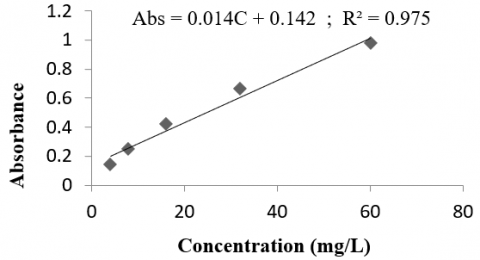Figure 1. Chromium (III) calibration curve tested

2.3 Equilibrium isotherms

Batch mode adsorption tests have been performed on Chromium to examine the impact of various parameters of operation.

In Erlenmeyer, batch equilibrium tests were carried out in flash 250 ml of Cr+3 ion solutions with different initial concentrations and the optimum adsorbent weight and optimum solution pH material.

The bottles were mixed for 2 hours at a fixed speed of 250 rpm in a rotating stirrer water bath.

The solutions were then filtrated, using the atomic-absorption spectrophotometer at 540 nm to evaluated the residual Cr +3 ion concentration in the filtrate. From the mass balance equation, the adsorption of metal ions to the adsorbent surface was measured at a specific time .

The quantity of chromium adsorbed by a unit of bentonite mass was measured using the formula below (2):

$\mathbf{q} \mathbf{t}=\frac{(\mathbf{C o}-\mathbf{C e}) \times \mathbf{V}}{\mathbf{m}}$   (2)

where, qt, C0, Ce, v and m are: the amount adsorbed at time t(mg/g) initial metal ion concentration (mg/L), equilibrium metal ion concentration (mg/L), volume of the solution (L) and mass of the adsorbent (g), respectively.

The results may be expressed in percentage removal as given by the following formula (3).

As given by the following formula (3), the results can be expressed in the percentage removal [21, 22].

$\mathrm{E}(\%)=\frac{(\mathrm{Co}-\mathrm{Ce})}{\mathrm{Co}} \times 100$   (3)

3. Results and Discussion

It is necessary to differentiate between the various components of clay mineral mixtures and to obtain information on their structure, composition and structural changes before testing the bentonite. For this reason, natural Algerian Bentonite (NAB) samples were passed to different techniques analysis as FTIR, XRD, and EDS for bentonite characterization.

3.1 FTIR analysis of natural Algerian bentonite

The study of raw bentonite by Fourier transform infrared spectroscopy (FTIR) was conducted out in the range of 400-4000 cm-1 (Figure 2).

## 2a.png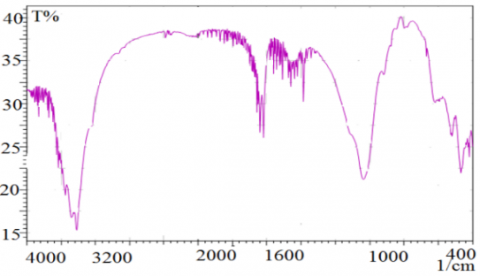(A)

## 2b.png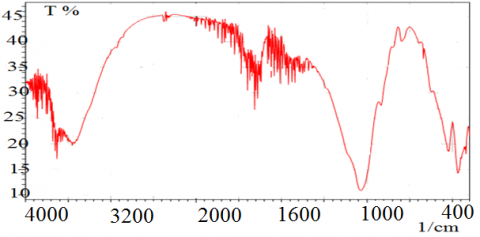(B)

Figure 2. FTIR spectra of (A) natural Algerian bentonite and (B) after adsorption

Vibrations of the OH groups for the water molecules adsorbed on the bentonite surface are responsible for the absorption bands of raw bentonite at 3627.60 cm-1. The band at 3411 cm-1 is due to OH- vibration of physically adsorbed water. The bending vibration mode of water is 1627 cm-1. The stretching vibration of the Si-O bands is attributed to the 1035 cm-1 band, and the 912 cm-1 band has been found to relate to the Al-Al-OH bending frequencies for 835 cm-1, the Al-Mg-OH bending vibrations were noted. The bands at 443 and 509 cm-1 relate to Al-O-Si and Si-O-Si bending vibrations, respectively.

In addition, the raw bentonite after adsorption (NAB-Cr(III)) sample spectrum shows that no structural change was observed in the clay sample after chromium adsorption , and only peak shifts and peak intensity changes were observed, there are many impurities for example chromium oxides and argillaceous complexes after adsorption.

3.2 XRD analysis

The X-ray diffraction (XRD) analysis for natural Algerian bentonite was used to identify the bentonite structure. Figure 3 shows the XRD spectrum for the natural Algerian bentonite before, and after adsorption.

The analysis of the spectrum of the raw bentonite used illustrates the following characteristic peaks: Quartz (2Ө=26.34), montmorillonite (2Ө=23.67, 2Ө=19.88), illite (2Ө=37).

The XRD pattern of natural Algerian bentonite charged by chrome after adsorption demonstrate the apparition of peaks related different crystalline chromium oxides (2Ө=53, 2Ө= 58, 2Ө= 68, 2Ө= 78).

## 3a.png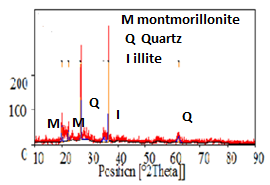(A)

## 3b.png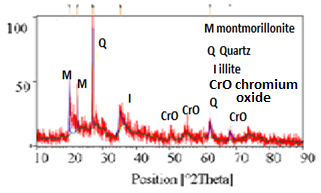(B)

Figure 3. XRD pattern of (A) natural Algerian bentonite and (B) after adsorption

3.3 MEB/EDX analysis of natural Algerian bentonite

The morphological analysis and elemental analysis of raw bentonite was prepared using an energy dispersive spectrometer (SEM/ EDX) scanning electron microscope.

Table 2. Elemental of analysis of natural Algerian bentonite

 Element Weight % Atomic % O 49.89 58.88 Si 15.19 10.22 C 11.77 18.50 Ca 9.23 4.35 Al 6.65 4.65 Fe 4.05 1.37 K 1.57 0.76 Mg 1.34 1.04 Na 0.26 0.21 Cu 0.05 0.02

## 4a.gif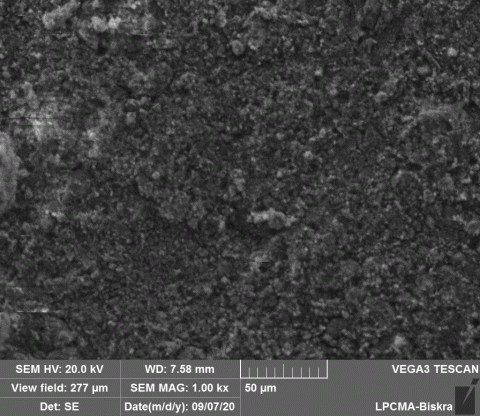(A)

## 4b.gif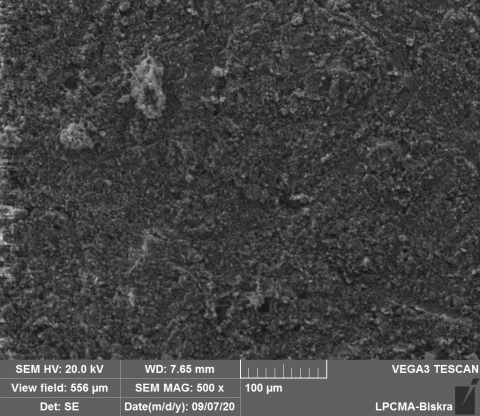(B)

## 4c.gif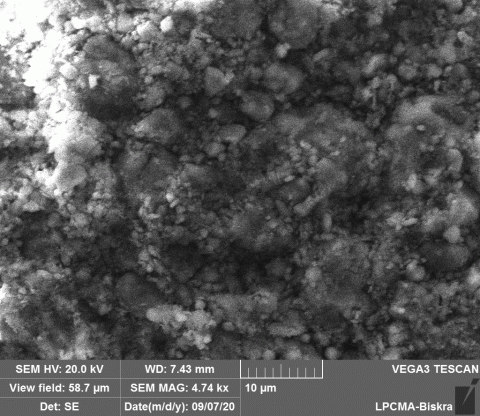(C)

Figure 4. SEM images of the natural Algerian Bentonite (A magnification: ×4.74k, B magnification: ×1.00k, C magnification: ×500)

To analyse the texture of the clay sample and to classify mineralogical assemblages, scanning electron microscopy (SEM) is used the Figure 4 obtained by scanning electron microscopy of the clay sample.

The findings of the scanning electron microscopy (SEM) study have shown that there is a morphological structure of natural Algerian bentonite in aggregates and in non-uniform surfaces.

The EDX analysis indicated that oxygen (49.89%) and silicon (15.19%) were major composition of natural bentonite (Table 2). In addition, C (11.77%), Ca (9.23), Al (6.65), Fe (4.05), K (1.57), Mg (1.34%), Na (0.26%) and Cu (0.05%).

3.4 pH point of zero charges (pH PZC)

The experimental protocol for determining the pH point of zero charge is as follows:

50 mg of the adsorbent is added to 50 ml of water at different pH ranging from 2to 12, the pH adjusted by adding HCl or NaOH to 0.01 M. The adsorbent mass is agitated for 24 hours.

Based on Figure 5, there is a quasi-stability of the final pH, in the initial pH range of 5 to 8, so the bearing formed by this constancy of the final pH can be related to the surface charge transfer, of the adsorbent from positive to negative and vice versa, pH pzc value found in this raw bentonite equal 6.4 ±0.1.

## 5.png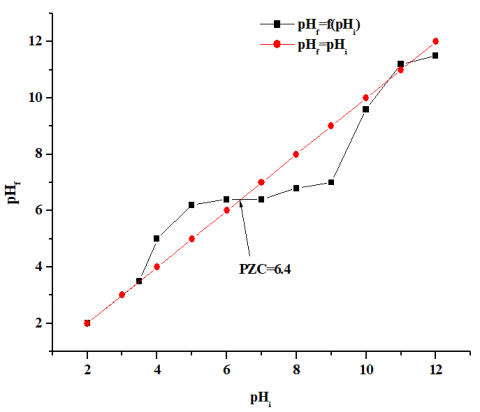Figure 5. pH point of zero charges (pH PZC)

3.5 The effect of the physicochemical parameters

The study of the adsorption of Cr+3 ions on natural bentonite requires knowledge of the parameters that influence this phenomenon, namely: Initial concentration, adsorbent dosage, pH, stirring time.

3.5.1 Influence of the initial concentration of Cr +3

We carried out this experiment for initial concentration Cr+3 ranging from 3 to 24 mg / l. The dose of the introduced bentonite is 1 g / l, pH=4.0 and temperature=18℃.

According to the results presented in Figure 6, we can see an increase in adsorption efficiency then a gradual decrease with the increase of the initial content of Cr+3, the increase of the initial trivalent chromium concentration would increase the driving force of mass transfer and thus the rate at which trivalent chromium ions move from the solution phase to the carbon surface.

The gradual decreasing explained of the saturation of active sites of the adsorbent.

In sodium chloride solution (salt water) the rate and capacity of trivalent chromium adsorption by natural bentonite are better compared to those obtained in the absence of NaCl.

This can be explained by the activation by Na+ ions of the sites of bentonite adsorption where new sites of adsorption would be created . With the initial concentration growing of Cr+3, this effect is intensified. It can be established that with the increase in the concentration of NaCl (ionic solution strength), the adsorption efficiency increases.

## 6.png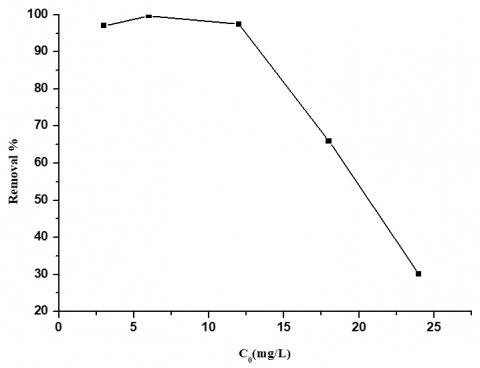Figure 6. The effect of the initial concentration of Cr +3 on the adsorption performance (m=1g/L, pH=4, T=18℃, agitation time=2h)

3.5.2 Effect of dosage of adsorbent

Figure 7 demonstrates the influence of the natural bentonite dosage on the adsorption mechanism.

Adsorption performance of different raw bentonite weights (25, 125, 250, 375, 500, 750 mg) combined with 250 ml of chromium solution at pH=4.

The consequence shows that the efficiency of adsorption increases slightly with bentonite dose before balance is achieved.

The improvement in the effectiveness of adsorption can be attributed to the increased abundance on the adsorbent surface of the number of active sites.

## 7.pngFigure 7. The influence of the amount of adsorbent on the efficiency of adsorption (C0=6mg/L, pH=4, T=18℃, agitation time= 2h)

At a bentonite dosage of 375 mg, the complete elimination occurs. After that, while any adsorbent has a finite number of active sites that are saturated with of active sites that are saturated with Cr3+ over time, adsorption is steady.

3.5.3 Effect of pH and adsorption mechanism

By varying the pH from 3 to 12, the effect of pH on the adsorption of Cr3+ ions were investigated. The adsorption percentage for raw bentonite rises in pH (3-6) is shown in Figure 8, It appears that the elimination rate of chromium ions is affected by the surface charges of the adsorbent, which are variable depending on the pH. For natural Algerian Bentonite (NAB) the highest efficiency (99.63%) was obtained at a pH - equal 6, this related to a decrease in competitiveness between H+ and Cr +3 and reduction in positive surface charge between the surface of bentonite particles and Cr+3 ions, the electrical charge is lower.

The slight reduction in the ability to remove Cr+3 ions at pH >6 may be due to the development of complexes between Cr3+ and OH-.

This hydroxyl chrome will take part in adsorption and precipate into the raw bentonite structure.

Adsorption and ion exchange are the fundamental mechanism. That control the adsorption characteristics of bentonite at pH ranging from 3 to 6. At these pH levels, exchangeable cations present at exchangeable sites (Na+, K+, Ca2 + and Mg +2 are exchanged for Cr +3 cations in aqueous solutions.

Several authors have mentioned the following mechanism for the adsorption of heavy metals at negative bentonite sites .

$\boldsymbol{M}^{+n}+\boldsymbol{H}_{2} \boldsymbol{O} \leftrightarrow \boldsymbol{M O H}^{+}+(\boldsymbol{n}-\mathbf{1}) \boldsymbol{H}^{+}$   (4)

$\mathbf{M O H}^{+}+\boldsymbol{X}^{-} \leftrightarrow \boldsymbol{X M O H}$  (5)

As shown in Eqns. (6) and (7) below the solution will cause the surface functional groups on clay particles to protonate and deprotonate by adsorption of OH- or H+ ions, respectively:

$\mathbf{X O H}+\boldsymbol{H}^{+} \leftrightarrow \boldsymbol{X}-\boldsymbol{O H}_{2}^{+}$   (6)

$\boldsymbol{X} \boldsymbol{O} \boldsymbol{H} \leftrightarrow \boldsymbol{X}-\boldsymbol{O}^{-}+\boldsymbol{H}^{+}$   (7)

X: surface of bentonite; M: metal, in our study M is the chrome (Cr).

The pH effect on the chromium ions adsorption by raw bentonite (NAB) can be explained on the basis of pHpzc, for which the charge of the adsorbent surface is positive lower than pHpzc of bentonite (pHpzc = 6.4±0.1). As the pH increase, the number of negatively charged sites augments and improves the chromium ions adsorption by electrostatic attractions.

The degradation of Cr(III) at pH above pH PZC is enhanced by the modifying the surface metal complexes in the ionized silanol and aluminol (Si-O-and Al-) groups, the complex Cr +3 reaction , and Cr (OH)3.

When Cr (VI) Oxyanions in solution are in contact with certain functions (-COOH,- OH) on the adsorption sites, they are typically minimized by specific reduction to less toxic Cr(III) cations, and raw bentonite has effectively adsorbed the lowered Cr(III).

As Cr (III) is insoluble at neutral and basic pH, its elimination is rather simple compared to Cr (VI) removal. Therefore, the removal or reduction of Cr (VI) to Cr (III) present as a key method for removal of Cr (VI) polluted water and waste water .

## 8.png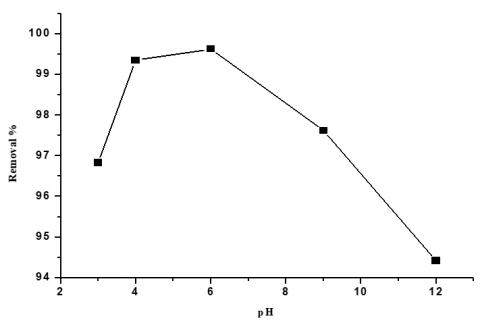Figure 8. The effect of pH on adsorption of Cr +3 on the adsorption performance (m=1g/L, C0=6mg/L, T=18℃, agitation time= 2h)

3.5.4 Effect of agitation time

We followed the adsorption kinetics of Cr+3 for an initial chromium content equal to 6 mg/l, a constant dosage of the adsorbent (1 g/l), pH = 4 and temperature is 18.8℃. the results obtained (Figure 9) show that the removal efficiency of Cr+3 varies with the stirring time, after 5 minutes, the maximum efficiency was achieved.

Indeed, for different stirring speeds (50, 150 and 250 rpm), the maximum value corresponding to the removal at this time is 92%, after which the adsorption rate becomes basically very slow.

The difference in the degree of adsorption may well be due to the fact that at the beginning of adsorption, all the sites on the surface of the adsorbent are free and the concentration gradient of the solute is extremely high.

Furthermore, the degree of removal of Cr+3 decreased with the increase in contact time, which refers to the number of vacant sites on the bentonite surface.

## 9.png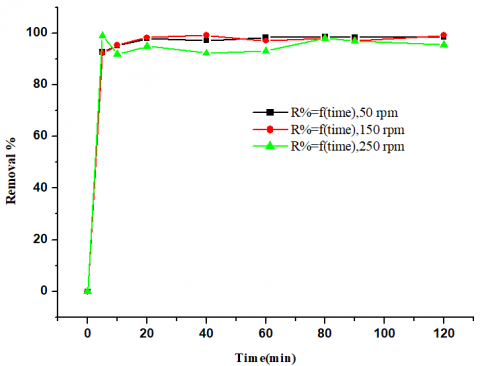Figure 9. The effect of agitation time of Cr+3 on the adsorption performance (C0=6mg/L, pH=4, T=18℃)

3.6 Adsorption isotherms of chrome ions on the bentonite

For the removal of Cr+3 on bentonite, the Langmuir and Freundlich adsorption isotherms using linear forms were also used as follows [25, 26].

Langmuir linear form:

$\frac{1}{q}=\frac{1}{q m b C e}+\frac{1}{q m}$  (8)

where, Ce is the equilibrium concentration in (mg/l), qm is the adsorption capacity calculation of the adsorbent (mg/g) and b is the Langmuir constant, which is the adsorption energy calculation (l/mg).

Freundlich linear form:

Ln $\mathbf{q e}=\operatorname{Ln} \mathbf{k}+\left(\frac{1}{\mathbf{n}}\right) \operatorname{LnCe}$  (9)

where, Ce is the concentration of equilibrium in (mg/l), qe is the amount of equilibrium adsorbed in (mg/g), K and 1/n are constants of Freundlich, respectively.

Considering the following operating conditions, we used the results according to the Freundlich and Langmuir model’s linear form. The initial chromium concentration is 6 mg/l, the bentonite mass used is 1g/l, the stirring speed is 250 rpm, pH=4, the contact time is 2 hours, and the temperature is 18℃.

The curves obtained with a better correlation coefficient showed that it can be estimated from our experimental conditions based on the correlation coefficient R2 (Table 3) that the Langmuir model is particularly suitable to the experimental data than the Freundlich model (Figure 10).

## 10a.png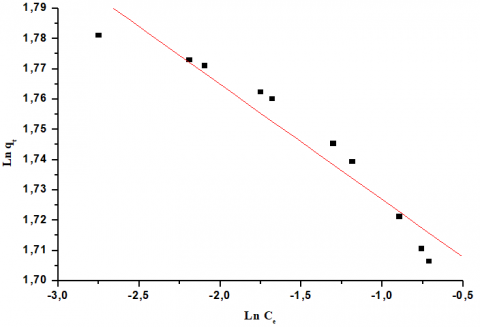(A)

## 10b.png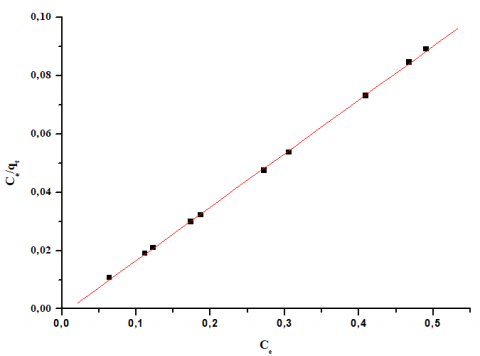(B)

Figure 10. Linear transformation of the adsorption Isotherm using (A) the Freundlich model, (B) The Langmuir model

Table 3. Values of the coefficients of the two linearized kinetic models with experimental results

 Langmuir Freundlich qm (mg/g) b (L/mg) RL R2 n K (mg/g) R2 5.46 183.15 0.0009 0.999 -33.33 5.47 0.881

3.7 Chrome adsorption kinetics

To determine the constants of adsorption rate, kinetic data were analysed using two kinetic models [27-29] namely: the model of Lagergren pseudo-first-order which is expressed as in Eq. (10):

$\log \frac{q e-q t}{q e}=-\frac{K_{1} \cdot t}{2.3}$  (10)

And the pseudo-second- order model which is expressed as in Eq. (11):

$\frac{t}{q t}=\frac{1}{2 K_{2} q \mathrm{e}^{2}}+\frac{t}{q e}$  (11)

The most typical data model was chosen on the basis of the coefficient of correlation R2, as shown in (Table 4) and (Figure 11).

Accordingly, the pseudo-second-order kinetic model is very appropriate for bentonite adsorption of chromium ions.

## 11.png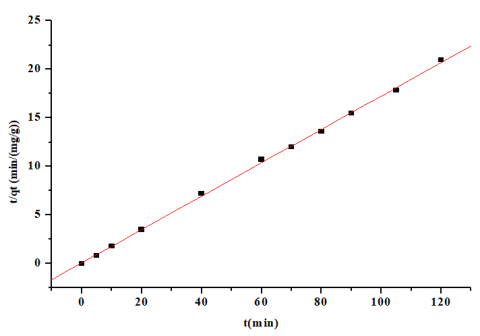Figure 11. Kinetics of the pseudo-second order

Table 4. Values of the coefficients of the two linearized kinetic models with experimental results

 Kinetics of the pseudo first order Kinetics of the pseudo-second order qe K1 R2 qe cal K2 h R2 - - 0. 044 5.84 0.0034 0.116 0.999

3.8 Comparison with various adsorbents in the literature

In order to give reason for the validity of the Natural Algerian bentonite (NAB) as a successful adsorbent for chromium ions charged in salt water, we compared our consequences with other works published elsewhere using different adsorbents and Table 5 gives a summary of maximum adsorption capacities of NAB on Cr (III) compared to different adsorbents.

Table 5 shows better value for (NAB) bentonite on Cr (III) adsorption, which the adsorption capacity is 5.46 (mg∙g−1) and the other adsorbents have the values 0.53 (mg∙g−1), 0.73 (mg∙g−1) for natural sepiolite and Kaolinite respectively.

Table 5. Adsorption capacity of Cr(III) by various adsorbents

 Adsorbent Heavy metal maximum adsorption capacities reference Natural sepiolite Cr+3 Qm (meq/g) 0.53  Kaolinite Cr+3 Qm (mg/g) 0.73  Bentonite GMZ Cr+3 Qm (mg/g) 4.68  Bentonite (NAB) Cr+3 Qm (mg/g) 5.46 This study
4. Conclusions

Batch adsorption experiments were conducted using natural Algerian Bentonite for the removal of Cr+3 from salt water and the findings obtained could also be summarized as follows:

Efficiency of removal of chromium ions increases with increases in the introduced mass of adsorbent, when the optimum dose (1.5 g/l) exceeds output (99.33%) and decreases progressively with the initial concentration of chromium rising.

The best performance at pH 4 and 6, with an elimination rate adequate to 100% and the dominant mechanism for the adsorption of trivalent chromium ions in salt water is cation exchange, the results of the adsorption kinetics confirm that the adsorption is fast and maximum efficiency (98.38%) reached after 5 minutes of adsorption.

The Langmuir equation has well defined equilibrium isotherms giving a maximum adsorption capacity of 5.46 mg / g at 18℃. The adsorption kinetics can be well described by the equation of the pseudo-second - order model.

Ion exchange, complex formation, and surface adsorption mechanism play an essential function in the adsorption method of natural Algerian bentonite (NAB).

Acknowledgment

The authors would like to acknowledge University of Mohammed khieder Biskra, for financial and instrumental supports.

Nomenclature
 b Affinity parameter of Langmuir (L/mg) BT Variation of adsorption energy (J. mol-1. g. mg-1) $C_{0}$ Initial solute concentration (mg/L) $C_{e}$ Concentration of solute at time t (mg/L) E Elimination rate (%) h Initial rate of adsorption(mg/g.min) k Freundlich adsorption capacity (mg/g) Kf Freundlich constants K L Langmuir adsorption constant (L/mg) KT Equilibrium constant (L. mg-1) K1 Rate constant of the pseudo first order adsorption (L·min−1) K2 Second order rate constant of the pseudo-order adsorption (g∙mg−1∙min−1) m Mass of the adsorbent (g) n Freundlich adsorption intensity qe Amount of solute adsorbed per unit weight of adsorbent at equilibrium (mg / g) qm Maximum capacity (mg / g) qt Amount adsorbed at time t (mg/g) R Elimination rate (%) RL Separation factor R2 Correlation coefficient t Time (mn) v Volume of the solution (L)
References

 Cefalu, W.T., Hu, F.B. (2004). Role of chromium in human health and in diabetes. Diabetes Care, 27(11): 2741-2751. https://doi.org/10.2337/diacare.27.11.2741

 Singh, R., Mondal, M.K. (2012). Kinetics thermodynamic and equilibrium study of Cr (VI) adsorption from aqueous solutions by NCL. Korean Journal of Chemical Engineering, 29(1): 1782-1787. https://doi.org/10.1007/s11814-012-0092-2

 Zouboulis, A.I., Kydrosk, K.A., Matis, K.A. (1995). Removal of hexavalent Chromium anions from solutions by pyrite fines. Water Research, 29(7): 1755-1760. https://doi.org/10.1016/0043-1354(94)00319-3

 Saroj, K.S., Braislav, P., Gary, A. (2008). Chromium removal from water: a review. Journal of Water Supply: Research and Technology-Aqua, 57(8): 541-553. https://doi.org/10.2166/aqua.2008.080

 Beszedits, S. (1988). Chromium Removal from Industrial Wastewaters. In: J. O. Nriagu and E. Nieboer, Eds., Chromium in the Natural and Human Environments, John Wiley, New York, 232-263.

 Calder, L.M. (1988). Chromium contamination of groundwater. Advances in Environmental Science and Technology, 20: 215-229.

 Sengupta, A.K., Clifford, D. (1986). Important process variables in chromate ion exchange. Environmental Science & Technology, 20(2): 149-155. https://doi.org/10.1021/es00144a006

 Berta, G., David, C., Inmacula, O. (2005). Removal and recovery of Cr (VI) from polluted ground waters: A comparative study of ion-exchange technologies. Water Research, 39(18): 4317-24. https://doi.org/10.1016/j.watres.2005.08.015

 Gunatilake, S.K. (2015). Methods of removing heavy metals from industrial wastewater. Journal of Multidisciplinary Engineering Science and Technology, 1(1): 12-18.

 Fu, F.L., Wang, Q. (2011). Removal of heavy metal ions from wastewaters: A review. Journal of Environmental Management, 92(3): 407-418. https://doi.org/10.1016/j.jenvman.2010.11.011

 Mahmous, T., Malik, S.A., Hussain, S.T. (2010). Biosorption and recovery of heavy metals from aqueous solution by Eichhornia crassipes (water hyacinth) ASH. Bioresources, 5(2): 1244-1256.

 Rauf, M., Iqbal, J., Ikram, M., Rauf, N. (2003). Adsorption studies of Ni (II) from aqueous solution onto bentonite. Journal of Trace and Microprobe Techniques, 21(2): 337-342. https://doi.org/10.1081/TMA-120020267

 Koyuncu, M. (2012). Adsorption of Cr (VI) From textile waste water by using natural bentonite. IIOAB Journal, 3(3): 1-4.

 Fadel, A., Nacef, S. (2017). Examination of an Algeria clay in the retention of Zinc ions charged in brackish water. Korean Chemical Engineering Research Journal, 55(5): 685-689. https://doi/10.9713/kcer.2017.55.5.685

 Ouakouak, A., Rihani, A., Youcef, L., Hamdi, N., Guergazi, S. (2020). Adsorption characteristics of Cu (II) onto CaCl2 pretrated Algerian bentonite. Material Research Express, 7(2): 1-9. https://doi.org/10.1088/2053-1591/ab5ee4

 Kim, T.K., Park, S., Cho, S.Y., Kim, H.B., Kang, Y., Kim, S.D., Kim, S.J. (2005). Adsorption of heavy metals by brewery biomass. Korean Journal of Chemical Engineering, 22(1): 91-98. https://doi.org/10.1007/BF02701468

 Sirirak, J., Worawannotal, N., Suwanchawalit, C., Chayabutra, S. (2020). Preparation and characterization of lake pigments from sappan wood using Thai local clays. Journal of Metals, Materials and Minerals, 30(1): 20-28. https://doi.org/10.14456/jmmm.2020.3

 Rao, B., Mohan, Rao, V.V. (2015). Thermodynamic and isothermal studies of congo red adsorption onto modified bentonite. Korean Chemical Engineering Research Journal, 53(6): 770-775. https://dx.doi.org/10.9713/Kcer.2015.53.6.1

 Onal, M. (2006). Physicochimical properties of bentonites: An averview. Communications Faculty of Sciences University of Ankara Series B, 52(2): 7-21.

 Grassi, M., Kaykioglu, G., Belgiorno, V., Lofrano, G., (2012). Removal of Emerging Contaminants from Water and Wastewater by Adsorption Process. Springer, New York.

 Oliveria, E.A., Montanher, S.F., Andrader, A.D., Nobrega, J.A., Rollemberg, M.C. (2005). Equilibrium studies for the sorption of chromium and nickel from aqueous solution using rice bran. Process Biochemistry, 40(11): 3485-3490. https://doi.org/10.1016/j.procbio.2005.02.026

 Bastan, T., Tabatabai, M.A. (1992). Effect of cropping systems on adsorption of metals by soils. Soil Sciences, 331-337.

 Boukerroui, A., Ouali, M. (2001). Activation of a bentonite by an ammonium salt; evolution of the exchange capacity and of the specific surface area. Annales de Chimie Science des Matériaux, 25(7): 583-590. https://doi.org/10.1016/S0151-9107(01)80013-1

 Vo, A.T., Nguyren, V.P., Ouakouak, A., Nieva, A., Doma Jr, B.T., Tran, H.N., Chao, H.P. (2019). Efficient removal of Cr (VI) from water by biochar and activated carbon prepared through hydrothermal carbonization and pyrolysis: Adsorption-coupled reduction mechanism. Water Journal, 11(6): 1-14. https://doi:10.3390/w11061164

 Sharma, S.K., Petrusevski, B., Amy, G. (2008). Chromium removal from water. Journal of Water Supply: Research and Technology, 57(8): 541-553. https://doi.org/10.2166/aqua.2008.080

 Ho, Y.S., McKay, G. (1998). Comparison of chemisorption kinetic models applied to pollutant removal on various sorbents. Process Safety and Environment Protection, 76(4): 332-340. https://doi.org/10.1205/095758298529696

 Mohammed-Azizi, F., Dib, S., Boufatit, M. (2013). Removal of heavy metals from aqueous solutions by Algerian bentonite. Desalination and Water Treatment, 51(22-24): 4447-4458. https://doi.org/10.1080/19443994.2013.770241

 Bhattacharyya, K.G., Gupta, S.S. (2008). Adsorption of a few heavy metals on natural and modified kaolinite and montmorillonite. Advences in Colloid and Interface Science, 140(2): 114-131. https://doi.org/10.1016/j.cis.2007.12.008

 Foo, K.Y., Hameed, B.H. (2010). Insights into the modeling of adsorption isotherm systems. Chemical Engineering Journal, 156(1): 2-10. https://doi.org/10.1016/j.cej.2009.09.013

 Kocaoba, S. (2009). Adsorption of Cd (II), Cr (III) and Mn (II) on natural sepiolite. Desalination, 244(1-3): 24-30. https://doi.org/10.1016/j.desal.2008.04.033

 Chantawong, V., Harvey, N., Bashkin, V. (2003). comparison of heavy metal adsorption by Thai kaolin and ballclay. Water Air Soil Pollution, 148(1): 111-125. https://doi.org/10.1023/A:1025401927023

 Chen, Y.G., He, Y., Ye, W.M., Lin, C.H., Zang, X.F., Ye, B. (2012). Removal of chromium (III) from aqueous solutions by adsorption on bentonite from Gaomiaozi, China. Environmental Earth Science, 67(1): 1261-1268. https://doi.org/10.1007/s12665-012-1569-3